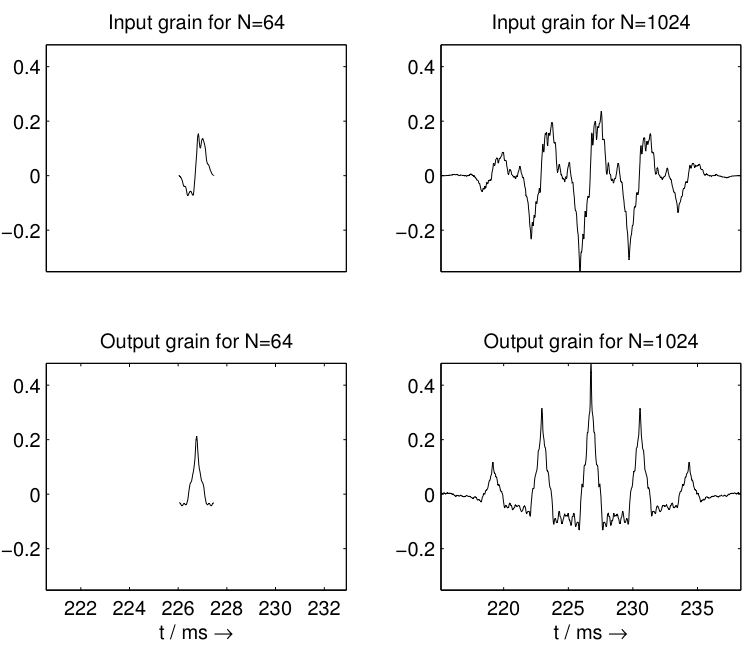Submitted by vincent on Fri, 08/07/2009 - 17:28
Author
Vincent Verfaille
Version
0.1

The robotization effect consists in transforming a human voice into a robot voice. One implementation is based on the sort-term Fourier transform (STFT), and replaces the sound by a series of symmetrical impulses, which spacing determines the robot fundamental frequency. In its adaptive version, robotization uses some sound descriptors to control the fundamental frequency and the roughness of the robot sound.

Implementation details
The overlap-add process of the short-term Fourier transform (phase vocoder without phase unwrapping nor frequency evaluation from the phase) is modified so as to independently control the window size N and the time increment T. Each grain is windowed (using a Hann periodic window), its STFT is then computed, and the phases are modified so that they become 0 in the center of the window. By aligning phases to the window ceter, it creates a peak in the middle of the window, which can also be seen as an impulse with the same frequency content as the original grain in terms of frequency and phase. The process is then repeated throughout the sound, with time increments computed so that two successively processed grains are separated by a number of samples that matches the ratio of the sampling rate and the target fundamental frequency. In between two processed grains, the remaining samples are simply to 0.Note that the magnitude spectrum is locally preserved where selected grains are processed, which means that the spectral envelope and its formants are locally preserved too.

Control parameters

• RA[n]: time increment between windows
• N[n]: window size

The robot voice's fundamental frequency F0[n] is locally defined a:
F0[n] = sampling rate / RA[n].
The value of N modifies the roughness of the sound as follows: the smaller N, the rougher the sound.
Here are two examples, for a small window/grain size (N=64 samples at 44.1kHz) on the left, and for a large window/grain size (1024 samples at 44.1kHz) on the right:Note that for higher window/grain sizes (N=1024 and above), the grain waveform presents an oscillation, which shows that the original fundamental frequency is getting more and more present. There is a trade-off to find between the roughness of the sound and the amount of original fundamental frequency to be heard. To start with, window/grain size of N=512 is good.

Perceptual viewpoints
The following attributes are modified:

• loudness (locally)
• pitch (via fundamental frequency, controlled by the time increment RA[n])
• timbre: roughness (via the window size N[n])
• timbre: harmonicity (2 pitches for large window size)

The following attributes are not modified:

• spectral envelope (at least locally)

Flowchart diagramBibliography